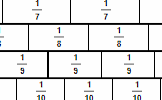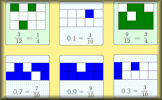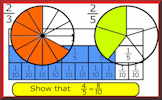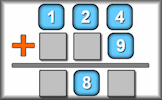#The objective of this challenge is to enter five fractions each one larger than the one before. The fraction to get you started is .

Enter a fraction less than one but greater than

0
0
.

Error Message

Enter a fraction less than one but greater than

0
0
.

Error Message

Enter a fraction less than one but greater than

0
0
.

Error Message

Enter a fraction less than one but greater than

0
0
.

Error Message

Enter a fraction less than one but greater than

0
0
.

Error Message

Enter a fraction less than one but greater than

0
0
.

Error Message

Enter a fraction less than one but greater than

0
0
.

Error Message

Enter a fraction less than one but greater than

0
0
.

Error Message

Enter a fraction less than one but greater than

0
0
.

Error Message

Enter a fraction less than one but greater than

0
0
.

Error Message

Enter a fraction less than one but greater than

0
0
.

Error Message

Enter a fraction less than one but greater than

0
0
.

Error Message

Well done. You have reached the end of this challenge

Congratulations. You have completed all of the challenges. There is a trophy waiting for you.

This activity comprises of  challenges each one requiring you to type in one more fraction than the previous challenge and each starting with a different fraction. The starting fractions are . A Transum Trophy is available for completing all of the challenges.

A vinculum is a mathematical symbol. It is a line that goes either above or below an expression or series of digits to show that they are grouped together. A vinculum servers the same purpose as a pair of brackets in many situations. The line drawn in the middle of a fraction has been called a vinculum by some which is convenient, particularly when considering the order of operations in an algebraic fraction or a fraction where the numerator or denominator is a calculation.

There are many more fraction activities, visual aids and lesson starters on our Fractions topic page and here are some recommended activities from that page.

#### Fraction WallThe traditional fraction wall diagram showing the relationship between simple fractions. This visual aid may help some pupils complete the Vinculum challenge.

#### Fraction LineArrange the fractions in order from smallest to largest. There are three different versions of this fraction ordering activity providing different levels challenge.

#### Equivalent FractionsPractise finding equivalent fractions numerically and in fraction diagrams. This is a classic exercise presented in three levels of difficulty.

## Transum.org

This web site contains over a thousand free mathematical activities for teachers and pupils. Click here to go to the main page which links to all of the resources available.## More Activities:

Mathematicians are not the people who find Maths easy; they are the people who enjoy how mystifying, puzzling and hard it is. Are you a mathematician?

Comment recorded on the 1 August 'Starter of the Day' page by Peter Wright, St Joseph's College:

"Love using the Starter of the Day activities to get the students into Maths mode at the beginning of a lesson. Lots of interesting discussions and questions have arisen out of the activities.
Thanks for such a great resource!"

Comment recorded on the 9 May 'Starter of the Day' page by Liz, Kuwait:

"I would like to thank you for the excellent resources which I used every day. My students would often turn up early to tackle the starter of the day as there were stamps for the first 5 finishers. We also had a lot of fun with the fun maths. All in all your resources provoked discussion and the students had a lot of fun."

Each month a newsletter is published containing details of the new additions to the Transum website and a new puzzle of the month.

The newsletter is then duplicated as a podcast which is available on the major delivery networks. You can listen to the podcast while you are commuting, exercising or relaxing.

Transum breaking news is available on Twitter @Transum and if that's not enough there is also a Transum Facebook page.

#### Nine DigitsArrange the given digits one to nine to make three numbers such that two of them add up to the third. This is a great puzzle for practicing standard pen and paper methods of three digit number addition and subtraction.

## Numeracy

"Numeracy is a proficiency which is developed mainly in Mathematics but also in other subjects. It is more than an ability to do basic arithmetic. It involves developing confidence and competence with numbers and measures. It requires understanding of the number system, a repertoire of mathematical techniques, and an inclination and ability to solve quantitative or spatial problems in a range of contexts. Numeracy also demands understanding of the ways in which data are gathered by counting and measuring, and presented in graphs, diagrams, charts and tables."

Secondary National Strategy, Mathematics at key stage 3

## Go Maths

Learning and understanding Mathematics, at every level, requires learner engagement. Mathematics is not a spectator sport. Sometimes traditional teaching fails to actively involve students. One way to address the problem is through the use of interactive activities and this web site provides many of those. The Go Maths main page links to more activities designed for students in upper Secondary/High school.

## Teachers

If you found this activity useful don't forget to record it in your scheme of work or learning management system. The short URL, ready to be copied and pasted, is as follows:

Do you have any comments? It is always useful to receive feedback and helps make this free resource even more useful for those learning Mathematics anywhere in the world. Click here to enter your comments.For All: